# Horizontal distribution of the zeros of $\zeta'(s)$

Can one use random matrix theory to predict the horizontal distribution of the real parts of the zeros of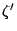? It is known that the Riemann Hypothesis is equivalent to the assertion that each non-real zero of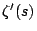has real part greater than or equal to 1/2. Moreover, if such a zero ofhas real part 1/2, then it is also a zero of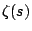(and so a multiple zero of). These assertions are the point of departure for Levinson's work on zeros of the Riemann zeta-function on the critical line [MR 54 #5135]. It would be interesting to know the horizontal distribution of these zeros; in particular what proportion of them with ordinates between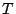and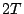are within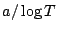of the 1/2-line? See the paper [ MR 98k:11119] of Soundararajan for the best theoretical result in this direction.

Back to the main index for L-functions and Random Matrix Theory.